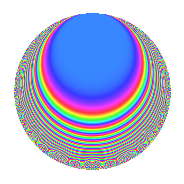# Properties

 Label 616.2.aLevel 616 Weight 2 Character orbit a Rep. character $$\chi_{616}(1,\cdot)$$ Character field $$\Q$$ Dimension 14 Newforms 8 Sturm bound 192 Trace bound 5

# Related objects

## Defining parameters

 Level: $$N$$ = $$616 = 2^{3} \cdot 7 \cdot 11$$ Weight: $$k$$ = $$2$$ Character orbit: $$[\chi]$$ = 616.a (trivial) Character field: $$\Q$$ Newforms: $$8$$ Sturm bound: $$192$$ Trace bound: $$5$$ Distinguishing $$T_p$$: $$3$$, $$5$$

## Dimensions

The following table gives the dimensions of various subspaces of $$M_{2}(\Gamma_0(616))$$.

Total New Old
Modular forms 104 14 90
Cusp forms 89 14 75
Eisenstein series 15 0 15

The following table gives the dimensions of the cuspidal new subspaces with specified eigenvalues for the Atkin-Lehner operators and the Fricke involution.

$$2$$$$7$$$$11$$FrickeDim.
$$+$$$$+$$$$+$$$$+$$$$1$$
$$+$$$$+$$$$-$$$$-$$$$4$$
$$+$$$$-$$$$+$$$$-$$$$2$$
$$+$$$$-$$$$-$$$$+$$$$1$$
$$-$$$$+$$$$-$$$$+$$$$2$$
$$-$$$$-$$$$+$$$$+$$$$1$$
$$-$$$$-$$$$-$$$$-$$$$3$$
Plus space$$+$$$$5$$
Minus space$$-$$$$9$$

## Trace form

 $$14q + 4q^{5} + 10q^{9} + O(q^{10})$$ $$14q + 4q^{5} + 10q^{9} + 6q^{11} - 4q^{13} + 4q^{15} + 4q^{17} - 12q^{23} + 6q^{25} + 12q^{27} - 4q^{29} - 4q^{31} + 24q^{37} - 8q^{39} - 12q^{41} - 8q^{43} + 32q^{45} + 8q^{47} + 14q^{49} - 8q^{51} - 4q^{53} - 8q^{59} + 20q^{61} - 8q^{63} + 32q^{65} - 4q^{67} - 12q^{69} + 12q^{71} - 12q^{73} + 12q^{75} - 4q^{77} - 24q^{79} + 14q^{81} + 16q^{83} - 56q^{87} - 16q^{89} + 4q^{91} - 4q^{93} - 32q^{95} - 32q^{97} + 18q^{99} + O(q^{100})$$

## Decomposition of $$S_{2}^{\mathrm{new}}(\Gamma_0(616))$$ into irreducible Hecke orbits

Label Dim. $$A$$ Field CM Traces A-L signs $q$-expansion
$$a_2$$ $$a_3$$ $$a_5$$ $$a_7$$ 2 7 11
616.2.a.a $$1$$ $$4.919$$ $$\Q$$ None $$0$$ $$-2$$ $$2$$ $$1$$ $$+$$ $$-$$ $$+$$ $$q-2q^{3}+2q^{5}+q^{7}+q^{9}-q^{11}+4q^{13}+\cdots$$
616.2.a.b $$1$$ $$4.919$$ $$\Q$$ None $$0$$ $$-1$$ $$-1$$ $$1$$ $$+$$ $$-$$ $$-$$ $$q-q^{3}-q^{5}+q^{7}-2q^{9}+q^{11}+q^{15}+\cdots$$
616.2.a.c $$1$$ $$4.919$$ $$\Q$$ None $$0$$ $$0$$ $$-2$$ $$1$$ $$-$$ $$-$$ $$+$$ $$q-2q^{5}+q^{7}-3q^{9}-q^{11}+2q^{13}+\cdots$$
616.2.a.d $$1$$ $$4.919$$ $$\Q$$ None $$0$$ $$0$$ $$0$$ $$-1$$ $$+$$ $$+$$ $$+$$ $$q-q^{7}-3q^{9}-q^{11}-6q^{13}-2q^{19}+\cdots$$
616.2.a.e $$1$$ $$4.919$$ $$\Q$$ None $$0$$ $$2$$ $$2$$ $$1$$ $$+$$ $$-$$ $$+$$ $$q+2q^{3}+2q^{5}+q^{7}+q^{9}-q^{11}+4q^{15}+\cdots$$
616.2.a.f $$2$$ $$4.919$$ $$\Q(\sqrt{17})$$ None $$0$$ $$-1$$ $$-3$$ $$-2$$ $$-$$ $$+$$ $$-$$ $$q-\beta q^{3}+(-2+\beta )q^{5}-q^{7}+(1+\beta )q^{9}+\cdots$$
616.2.a.g $$3$$ $$4.919$$ 3.3.229.1 None $$0$$ $$1$$ $$1$$ $$3$$ $$-$$ $$-$$ $$-$$ $$q-\beta _{2}q^{3}-\beta _{2}q^{5}+q^{7}+(1-\beta _{1})q^{9}+\cdots$$
616.2.a.h $$4$$ $$4.919$$ 4.4.11348.1 None $$0$$ $$1$$ $$5$$ $$-4$$ $$+$$ $$+$$ $$-$$ $$q+\beta _{2}q^{3}+(1-\beta _{1})q^{5}-q^{7}+(2+\beta _{2}+\cdots)q^{9}+\cdots$$

## Decomposition of $$S_{2}^{\mathrm{old}}(\Gamma_0(616))$$ into lower level spaces

$$S_{2}^{\mathrm{old}}(\Gamma_0(616)) \cong$$ $$S_{2}^{\mathrm{new}}(\Gamma_0(11))$$$$^{\oplus 8}$$$$\oplus$$$$S_{2}^{\mathrm{new}}(\Gamma_0(14))$$$$^{\oplus 6}$$$$\oplus$$$$S_{2}^{\mathrm{new}}(\Gamma_0(44))$$$$^{\oplus 4}$$$$\oplus$$$$S_{2}^{\mathrm{new}}(\Gamma_0(56))$$$$^{\oplus 2}$$$$\oplus$$$$S_{2}^{\mathrm{new}}(\Gamma_0(77))$$$$^{\oplus 4}$$$$\oplus$$$$S_{2}^{\mathrm{new}}(\Gamma_0(88))$$$$^{\oplus 2}$$$$\oplus$$$$S_{2}^{\mathrm{new}}(\Gamma_0(154))$$$$^{\oplus 3}$$$$\oplus$$$$S_{2}^{\mathrm{new}}(\Gamma_0(308))$$$$^{\oplus 2}$$